Courses

# Previous Year Questions - Introduction to Trigonometry Class 10 Notes | EduRev

## Class 10 : Previous Year Questions - Introduction to Trigonometry Class 10 Notes | EduRev

The document Previous Year Questions - Introduction to Trigonometry Class 10 Notes | EduRev is a part of the Class 10 Course Mathematics (Maths) Class 10.
All you need of Class 10 at this link: Class 10

Short Answer type Questions

Q 1. The ratio of the height of a tower and the length of its shadow on the ground is √ 3 : 1. What is the angle of elevation of the sun?   [Delhi 2017]
Ans: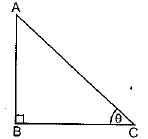Let, AB = height of the tower
BC = length of the shadow
∠ACB = θ = Angle of elevation of the sum
In ∠ABC, ∠B = 900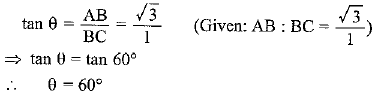Q 2.  An observer, 1.5 m tall, is 28.5 m away from a 30 m high tower. Determine the angle of elevation of the top of the tower from the eye of the observer.   [Delhi 2017 (C)]
Ans:   Draw AM || BD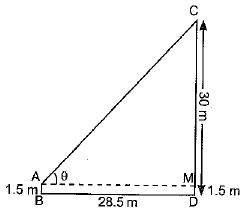Here, AB - DM - 1 . 5 m
CM = CD - DM

= 30 - 1.5 = 28.5 m
Let θ be the angle of elevation of the top of the tower from the eye of the observer
∴ In  ΔACM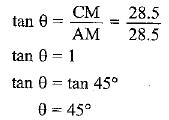Q 3.  If a tower 30 m high, casts a shadow 10√ 3 m long on the ground, then what is the angle of elevation of the sun   [AI 2017]
Ans:

Let θ be the angle of elevation of the sun
∴ In right angled triangle ABC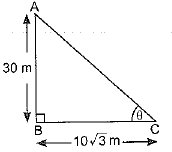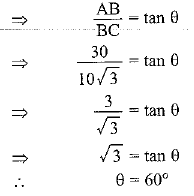Hence, the angle of elevation of the sun is 600.

Q 4. A moving boat is observed from the top of a 150 m high cliff moving away from the cliff. The angle of depression of the boat changes from 60° to 45° in 2 minutes. Find the speed of the boat in m/min. [Delhi 2017]
Ans:

Observe that AD is a transversal to the parallel lines AE and BD. Therefore ∠EAD and ∠ADB are alternate angles and so both are equal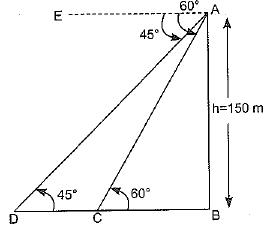So, ∠ADB = 45°
Similarly, ∠ACB = 60°
Let AB be the cliff.

∴ AB = 150 m
After 2 minutes it reaches at D.
In right triangle ABC,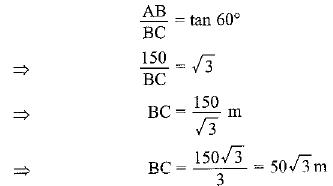In right triangle ABD,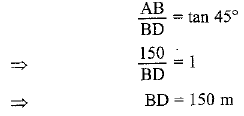Distance covered in 2 minutes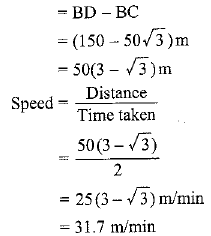Hence, speed of boat is 31.7 m/min

Q 5. A ladder 15 m long makes an angle of 60° with the wall. Find the height of the point where the ladder touches the wall.   [CBSE(F) 2017]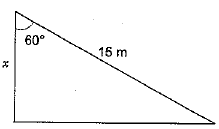Ans: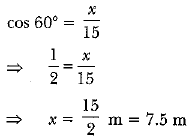Q 6. The ratio of the height of a tower and the length of its shadow on the ground is √3 : 1. What is the angle of elevation of the sun?    [CBSE Delhi 2017]
Ans: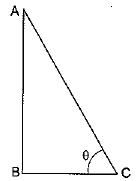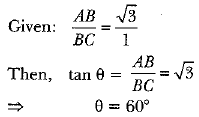Q 7. If a tower 30 m high, casts a shadow 10 √3 m long on the ground, then what is the angle of elevation of the sun? [CBSE (Al) 2017]
Ans:
In ΔABC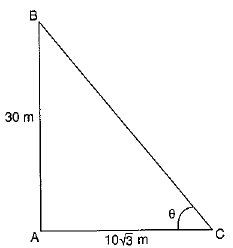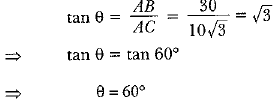Q 8. A moving boat is observed from the top of a 150 m high cliff moving away from the cliff. The angle of depression of the boat changes from 60° to 45° in 2 minutes. Find the speed of the boat in m/h.    [CBSE Delhi 2017]
Ans: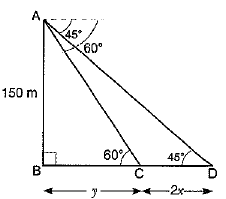Let the speed of boat be x m/min
∴ CD = 2x
In ΔABC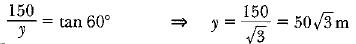In ΔABD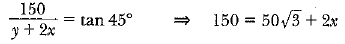(∵ y = 50√3)
⇒ x = 25(3-√3)
speed = 25(3-√3)m/min
∴ = 25 x 60 (3-√3)m/h = 1500(3-√3)m/h

Q 9. In given Figure, AB is a 6 m high pole and CD is a ladder inclined at an angle of 60° to the horizontal and reaches up to a point D of pole. If AD = 2.54 m, find the length of the ladder. (Use √3 =1.73)  [CBSE Delhi 2016]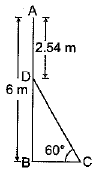Ans:

DB = (6 - 2.54)m = 3.46 m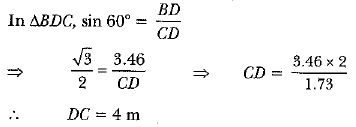Q 10. An observer, 1.7 m tall, is 20 √3 m away from a tower. The angle of elevation from the eye of observer to the top of tower is 30°. Find the height of tower.    [CBSE (F) 2016]
Ans: Let AB be the height of tower and DE be the height of observer.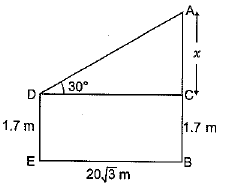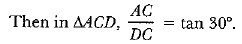⇒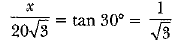⇒ x = 20m
∴ AB = 20 + 1.7 = 21.7m

Q 11. A vertical tower stands on a horizontal plane and is surmounted by a flagstaff of height 5 m. From a point on the ground the angles of elevation of the top and bottom of the flagstaff are 60° and 30° respectively. Find the height of the tower and the distance of the point from the tower. (Take √3 = 1.732)     [CBSE (F) 2016]
Ans: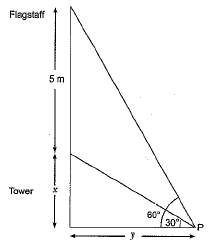Let height of tower be x m an d distance of point from tower be y m.
(i) from the fig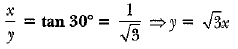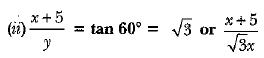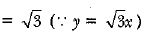⇒ x + 5 = 3x ⇒ x = 5/2 = 2.5
Height of tower = 2.5 m
Distance of point from tower = y = √3x
= (2.5 x 1.732) or 4.33 m

Q 12. Two men on either side of a 75 m high building and in line with base of building observe the angle of elevation of the top of the building as 30° and 60°. Find the distance between the two men. (Use √3 = 1.73)    [CBSE (F) 2016]
Ans: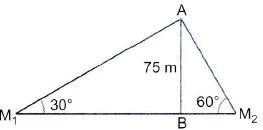ln ΔABM1,

Let AB be the building having height 75 m and the angles of elevation are 30° and 60° from the point M1 and M2 respectively;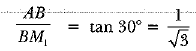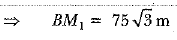In ΔABM2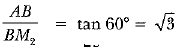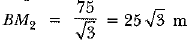∴ M1M2 = M1B + BM2
= 75√3 + 25√3 = 100√3m = 173m
∴ Distance between two men = 173 m.

Long Answer Type Questions

Q 1. Two poles of equal heights are standing opposite to each other on either side of the road, which is 80 m wide. From a point between them on the road, the angles of elevation of the top of the poles are 60° and 30°, respectively. Find the height of the poles and the distances of the point from the poles
[NCERT; CBSE 2019 (30/1/2)]
Ans: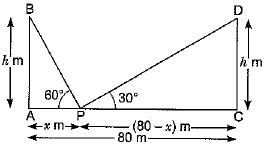∠APB = 60° and ∠DPC = 30°
The distance between two poles is 80m. (Given)
Let AP = x m, then PC = (80 - x) m
Now, in ΔAPB, we have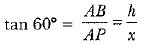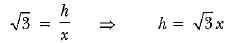...(i)
Again in ΔCPD, we have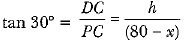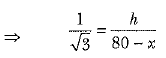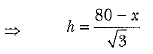...(ii)
From (i) and (ii), we have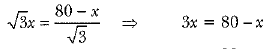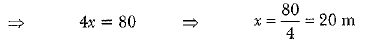Now, putting the value of x in equation (i), we have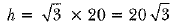Hence , the height of the pole is 20√3 m and the distance of the point from first pole is 20m and that of the second pole is 60 m.

Q 2. As observed from the top of a 100 m high light house from the sea-level, the angles of depression of two ships are 30° and 45°. If one ship is exactly behind the other on the same side of the light house, find the distance between the two ships. [Use√3 = 1.732]    [CBSE 2018]
Ans:  Let AB be the height of the light house from the sea-level. C and D are the two ships. One ship is exactly behind each other such that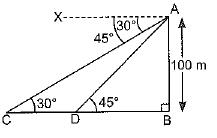∠XAC = ∠ACB

= 30° and ∠XAD
= ∠ADB = 45°

We have to find distance between two ships, i.e. CD
In ΔABC, ∠B - 90°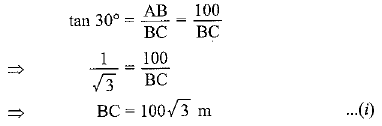Also, in Δ ABD, ∠B = 90°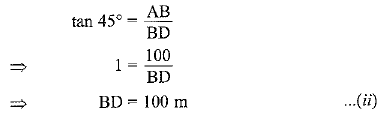From (i), we have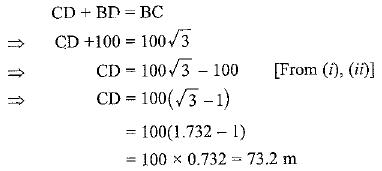Q 3. The angle of elevation of the top of a hill at the foot of a tower is 60° and the angle of depression from the top of tower to the foot of hill is 30°, If tower is 50 metre high, find the height of the hill.   [Delhi 2017, CBSE 2018 (C)]
Ans: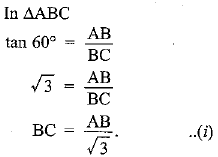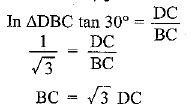Equating (i) and (ii), we get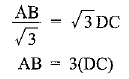Put DC = 50 m
AB = 3 x 50 = 150 m
Height of the hill = 150 m

Q 4. An aeroplane is flying at a height of 300 m above the ground. Flying at this height, the angles of depression from the aeroplane of two points on both banks of a river in opposite direction are 45° and 60° respectively. Find the width of the river.    [Use √ 3 = 1.732] [A1 2017]
Ans:

Let A be the position of the aeroplane and let C and D be two points on the two banks of the river such that the angles of depression at C and D are 60° and 45° respectively.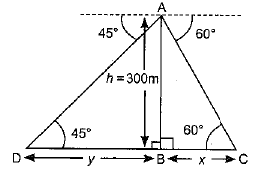Let    BC = x metres
and    BD = y metres
We have to find CD i.e. (x + y)m
In right angled triangle ABC.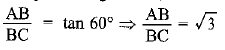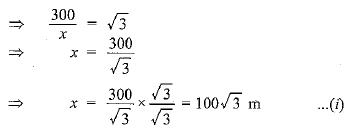In right angled triangle ABD,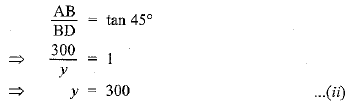From equations (i) and (ii), we get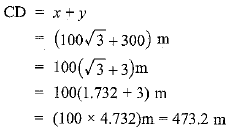Hence, the width of the river is 473.2 metres.

Q 5.  From a point P on the ground, the angle of elevation of the top of a 10 m tall building and a helicopter, hovering at some height vertically over the top the building are 30° and 60° respectively. Find the height of the helicopter above the ground.     [AI2017 (C)]
Ans: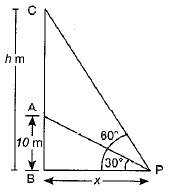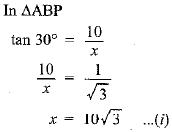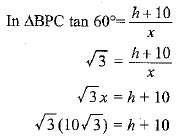[From (i)]
30 = h + 10
h = 20 m
The height of helicopter above the ground is 20 m.

Q 6. Amit, standing on a horizontal plane finds a bird flying at a distance of 200 m from him at an elevation of 30°. Deepak standing on the roof of a 50 m high building, finds the angle of elevation of the same bird to be 45°. Amit and Deepak are on opposite sides of the bird. Find the distance of bird from Deepak.   [CBSE, Allahabad 2019]
Ans: Given: Amit is standing at a distance of 200m from the bird flying at an elevation of 30°. Deepak is standing on the roof of 50m high building finds the angle of elevation of the bird to be 45°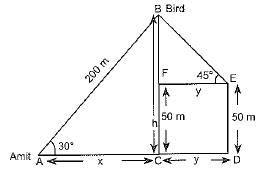Amit and Deepak are on opposite sides of the bird
To find: Distance between bird and Deepak i.e., BE.
Sol:  In ΔACB,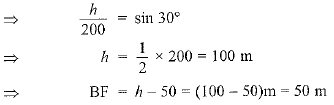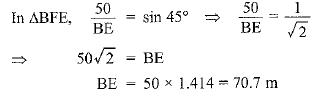Q 7. A bird is sitting on the top of a tree, which is 80 m high. The angle of elevation of the bird, from a point on the ground is 45°. The bird flies away from the point of observation horizontally and remains at a constant height. After 2 seconds, the angle of elevation of the bird from the point of observation becomes 30°. Find the speed of flying of the bird.    [Delhi 2016]
Ans:
Let bird is at A and after 2 seconds it reaches at E.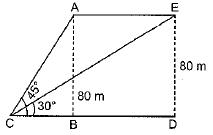∴  Distance covered = AE.
In right ΔABC,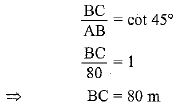In right ΔEDC,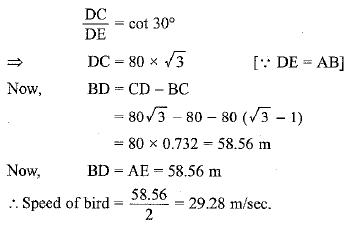Q 8. From the top of a 7 m high building, the angle of elevation of the top of a cable tower is 60° and the angle of depression of its foot is 45°. Determine the height of the tower.   [CBSE Delhi 2017]
OR
From the top of a 7 m high building, the angle of elevation of the top of a tower is 60° and the angle of depression of its foot is 45°. Find the height of the tower. [Use √3 = 1.732]     [CBSE (F) 2017]
Ans:
Let PQ be the building of height 7 metres and AB be the cable tower. Now it is given that the angle of elevation of the top A of the tower observed from the top P of building is 60° and the angle of depression of the base B of the tower observed from P is 45°.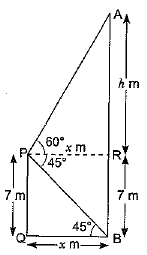So, ∠APR = 60° and ∠QBP = 45°
Let QB = xm , AR = h m, then , PR = x m
Now, in ΔAPR, we have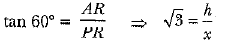⇒ √3x = h    ⇒ h = √3x     ..(i)
Again, in ΔPBQ we have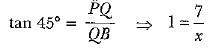⇒ x = 7       ..(ii)
Putting the value of x in equation (i), we have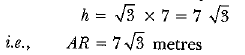So, the height of tower = AB = AR + RB = 7√3 + 7 = 7(√3+1)m.

Q 9. A straight highway leads to the foot of a tower. A man standing at the top of the tower observes a car at an angle of depression of 30°, which is approaching the foot of the tower with a uniform speed. Six seconds later, the angle of depression of the car is found to be 60°. Find the time taken by the car to reach the foot of the tower from this point.     [CBSE Delhi 2017 (C)]
Ans:

Let OA be the tower of height h, and P be the initial position of the car when the angle of depression is 30°.
After 6 seconds, the car reaches to Q such that the angle of depression at Q is 60°. Let the speed of the car be v metre per second. Then,
PQ = 6v     (∵ Distance  = speed x time)
and let the car take t seconds to reach the tower OA from Q as shown in figure. Then, OQ = vt metres. Now, in ΔAQO, we have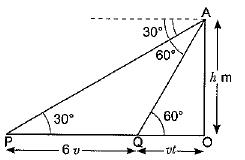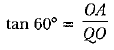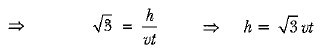... (i)
Now, in ΔAPO, we have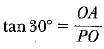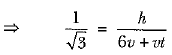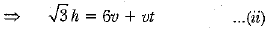Now, substituting the value of h from (i) into (ii), we have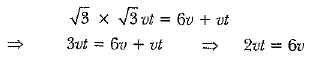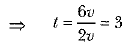Hence, the car will reach die tower from Q in 3 seconds.

Q 10. From the top of a tower, 100 m high, a man observes two cars on the opposite sides of the tower and in same straight line with its base, with angles of depression 30° and 45°. Find the distance between the cars. [Take S = 1.732]    [CBSE (AI) 2017]
Ans:
Let AO be the tower of height 100 m. Car B and Car C are in opposite direction and at distance of jc m and y m respectively.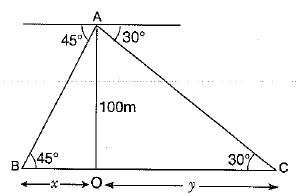In ΔABO,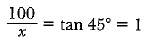⇒ x = 100
In ΔACO,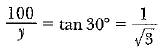⇒ y = 100√3
Distance between the cars - x + y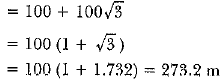Offer running on EduRev: Apply code STAYHOME200 to get INR 200 off on our premium plan EduRev Infinity!

## Mathematics (Maths) Class 10

51 videos|346 docs|103 tests

,

,

,

,

,

,

,

,

,

,

,

,

,

,

,

,

,

,

,

,

,

;